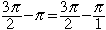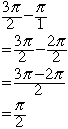Name: Karen Question: Hi I need to simplify this a) 3pi/2 - pi the example is this : pi - pi/3 =3pi/3 - pi/3 =2pi/3 The answer to a) is pi/2, I just can't figure out why. Thanks. Hi Karen, You are to subtract pi from 3pi/2. If you think of pi as the fraction pi/1 then the problem is to subtract two fractionsTo add or subtract two fractions you need to ensure that they have the same denominator. If you multiply the numerator and denominator of the second fraction by 2 then both fractions will have a denominator of 2 and you can subtract them.Cheers, Penny Go to Math Central